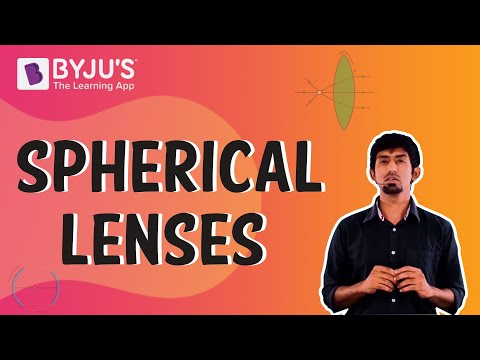# Biconvex Lens Questions

## Biconvex Lens

Biconvex lens is a simple lens that includes two convex shells in spherical form, with generally an equivalent kind of radius of curvature. These lenses are also named convex lenses.

The biconvex lens has a varied range of applications like control and focusing of laser beams, quality imaging and also the other kinds of optical instruments.

In a plano-convex lens, also known as a biconvex lens, a precisely parallel beam of light passes through the lens, which eventually converges it at a spot or focus behind the lens. Hence, for this reason this lens is identified as a converging or positive lens. The distance between the pole that is the centre point of the lens and the focus is termed as focal length. There exists two centres and two focal points in a biconvex lens because there is a curvature on both sides of the lens.

## Important Questions on Biconvex Lens

1) What are the types of convex lens?

The convex lens is fundamentally of three types, that is, the plano-convex lens, a double convex lens, and concave-convex lens.

2) What will be the nature of the image formed when an object is positioned at a distance of 30 cm from a convex lens of focal length 15 cm?

Here, u = -30cm and f = 15cm

According to the lens formula = (1/v-1/u = 1/f)

= 1/v – 1/ (-30) = 1/15

= v = 30cm

The image will be real and inverted at a distance of 30 cm on the right side of the lens.

3) In order to get a magnification of 1, at what distance from a converging lens of focal length 16 cm must an object be placed?

Magnification for converging lenses is 1 when the object is placed at 2F or C.

Since c=2×F

=2×16=32

So, u=32cm

4) What is a concave-convex lens?

A concave-convex lens is a combination of a lens one convex one concave lens side, that is concave-convex lens or meniscus. It is a lens that is curved outwards from one side and inwards from another side. This lens is used to control the laser beam. Also, it is used to balance out the spherical aberrations produced by other lenses.

5) What are the uses and applications of biconvex lenses?

Some of the important uses of biconvex lens are listed below-

1. Biconvex lenses are also used as condensing or magnifying lenses.
2. Biconvex lenses are also used as objectives or magnifiers
3. Imaging systems such as microscopes, eyeglasses, telescopes, projectors, cameras, etc. also utilize biconvex lenses.
4. In case of films that are photographic or an optical sensor, biconvex lenses are used in producing the virtual images.
5. Biconvex lenses are also used as a burning-glass.
6. In various kinds of industries, biconvex lenses are also used in the applications of image relays.

6) What is a double convex lens?

A double convex lens is a lens curved outwards from both sides, which is also termed as the biconvex lens. These lenses have a smaller focal length of equal diameter and surface radius. Double convex lenses are used for projectors, telescopes, etc. It produces real images for photography and virtual images for the human eye.

7) An object is placed at a distance of 100 cm from a converging lens of focal length 40 cm. What is the nature and position of the image?

Since the object is placed at a distance greater than the focal length of the lens, the image formed is real and inverted.

(ii) u=100cm, f=40cm

Lens formula:

1/v-1/u = 1/f

1/v – 1/(-100) = 1/40

v=66.6cm

Therefore, the image is formed 66.6cm behind the convex lens .

8) What are the rules for obtaining images formed by a convex lens?

Rule 1: If a ray of light is parallel to the principal axis initially, then, after refraction through the lens it passes through the focus.

Rule 2: A ray of light does not bend after refraction when it passes through the optical centre of the convex lens, it goes straight.

Rule 3: When the ray of light passes through the focus of the convex lens, then after refraction through the lens a ray of light becomes parallel to the principal axis.

9) Why is a convex lens termed a converging lens?

A convex lens is termed as a converging lens because it unites a parallel beam of light on a point which is known as principal focus.

10) Define focal length of a convex lens.

The focal length of a convex lens is defined as the distance between the centre of the lens and its focus, that is the point where the parallel rays converge.

### Practice Questions

1. What are the properties of biconvex lenses?
2. Define magnification of convex lens.
3. An erect image 3cm high is formed 15cm from a lens, the object being 0.5cm high. Determine the focal length of the lens.
4. What are the functions of convex lenses?
5. Differentiate between convex lens and concave lens.

 Related Articles

## Suggested VideosStay tuned to BYJU’S and Fall in Love with Learning!